## Introduction

The USGS NEIC provides Finite Fault Models for larger earthquakes. An example of this is at http://earthquake.usgs.gov/earthquakes/eqinthenews/2012/usc000cfsd/finite_fault.php.

This tutorial shows how to use the Computer Programs in Seismology codes to compute the static deformation for a given finite fault model.

## Theory

The solution for static deformation in a wholespace, halfspace and a layer isotropic media are given in the file static.pdf.
Two program are used: hsanal96 and hstat96. The first is used for the special problem of an observation point anywhere within an isotropic halfspace or at the surface of an isotropic halfspace. The program is an implementation of the close-form solutions given in the PDF document. The second program, hstat96, is much more general and will permit a source and observation point anywhere within a stack of isotropic layers with boundary conditions of halfspace, free or rigid at the top and bottom interfaces. hstat96 is essentially the limiting case of hspec96 as angular frequency goes to zero. Because of its generality, the execution time of  hstat96 is greater because of the multiplication of propagator matrices and the numerical integration over wavenumber.

The sequence of running these two programs is as simple as running   hspec96:

`hprep96 -M \${MODEL} -d dfile -ALL -HS \${EVDP} -HR 0 -TF -BHhsanal96hprep96 -M \${MODEL} -d dfile -ALL -HS \${EVDP} -HR 0 -TF -BHhstat96`
which is the sequence same sequence as for running hspec96. There are two differences though. First the hspec96 creates the output file hspec96.grn which is then convolved with the source time function to create the ASCII file in the file96 format, e.g.,
`hpulse96 -p -V -l 1 > file96`
Since the static deformation is just a single point, the hstat96 and hsanal96  directly create an output named file96. The other difference is that both programs accept a -V command line flag. The interesting part of the output is the following:

 hsanal96 hstat96 ` TSTATIC: 1 ZDD 1 -1.41254714E-06 TSTATIC: 2 RDD 2 -2.82509382E-06 TSTATIC: 3 ZDS 3 -4.84096199E-06 TSTATIC: 4 RDS 4 -9.68192307E-06 TSTATIC: 5 TDS 13 -1.31267000E-13 TSTATIC: 6 ZSS 5 2.73420915E-06 TSTATIC: 7 RSS 6 9.77280888E-06 TSTATIC: 8 TSS 14 -1.92498214E-06 TSTATIC: 9 ZEX 7 2.01586749E-06 TSTATIC: 10 REX 8 4.03173499E-06 TSTATIC: 11 ZVF 9 -1.71420732E-04 TSTATIC: 12 RVF 10 -7.14882117E-05 TSTATIC: 13 ZHF 11 9.19448121E-06 TSTATIC: 14 RHF 12 1.97109504E-04 TSTATIC: 15 THF 15 -1.35676630E-04` ` HSTATIC: 1 ZDD 1 -1.41254611E-06 HSTATIC: 2 RDD 2 -2.82509268E-06 HSTATIC: 3 ZDS 3 -4.84096336E-06 HSTATIC: 4 RDS 4 -9.68191762E-06 HSTATIC: 5 TDS 13 1.58707737E-13 HSTATIC: 6 ZSS 5 2.73421119E-06 HSTATIC: 7 RSS 6 9.77280979E-06 HSTATIC: 8 TSS 14 -1.92498396E-06 HSTATIC: 9 ZEX 7 2.01586795E-06 HSTATIC: 10 REX 8 4.03173590E-06 HSTATIC: 11 ZVF 9 -1.71379419E-04 HSTATIC: 12 RVF 10 -7.14995622E-05 HSTATIC: 13 ZHF 11 9.18313890E-06 HSTATIC: 14 RHF 12 1.97061323E-04 HSTATIC: 15 THF 15 -1.35628448E-04`

The purpose of this output is to compare the analytic results on the left to the numerical integration results on the right for each of the basic Greens functions. This test case was for a source at a depth of 20 km, a receiver at the surface and a distance of 20 km for a halfspace model with Vp=6.15 km/s, Vs=3.55 km/s and density 2.8 gm/cc.

Top of Page

To run the simulations, you will need the following:
• A velocity model in the CPS model96 format.
• the finite fault model given by a link at the bottom of the USGS Finite Fault solution page
• , and
• the archive DOSTATIC.tgz

Top of Page

## Setting up the test runs

Unpack the DOSTATIC.tgz using the commands:

```	gunzip -c DOSTATIC.tgz | tar xvf -
```

This will create the following directory structure:

```STATIC_TEST-|
|-FINITE_1
|
|-FINITE_2
|
|-FINITE_3
|
|-FINITE_4
```
In each sub-directory there will be an executable shell script named DOIT. This shell script computes the static deformation of a finite fault model and then plots a contour map.

The individual test cases differ in the following way:

• Finite_1: Use a simple halfspace model and the analytic solution for a halfspace (hsanal96)
• Finite_2: Use a simple halfspace model and the general wavenumber integration code (hstat96)
• Finite_3: Use the WUS model and the general wavenumber integration code (hstat96)
• Finite_4: Use the upper 120 km of AK135 and the general wavenumber integration code (hstat96)

These cases test the code and also investigate the sensitivity of the permanent displacements to the velocity model used.

Top of Page

## Requirements

• The latest version of Computer Programs in Seismology. Specifically you will need gsac, hstat96 and hsanal96.
• The following GMT programs: xyz2grd, surface, grdcontour, pscoastand pstext. You can edit the script to remove the contour plotting if you do not want to make plots.

Top of Page

## DOIT

Each of the directories that are unpacked contain a script named DOIT.  Follow  the link to an annotated discussion of the script DOIT.

Top of Page

## Example

The example uses the finite fault solution for the M7.7 - 83km E of Khash, Iran (2013-04-16 10:44:20 UTC) earthquake.  We use the "Subfault Format" as the data file for the simulation. The following three figures present the finite fault solution (left) and the projection of the slip distribution onto topography (center) and  INSAR image (right).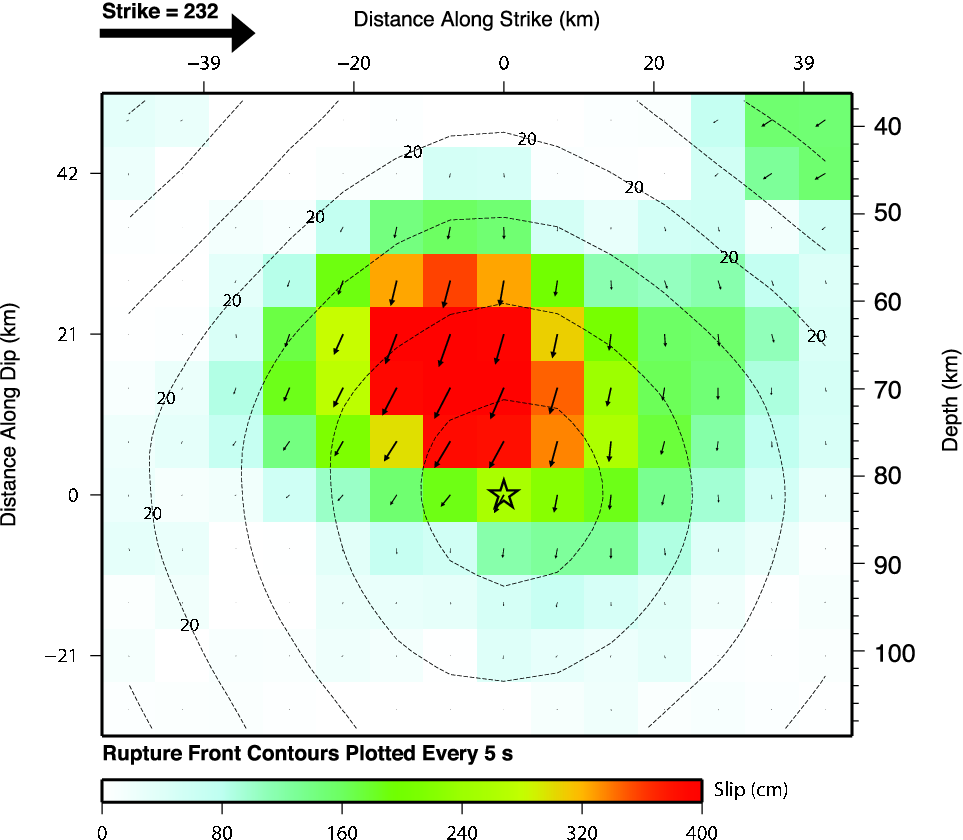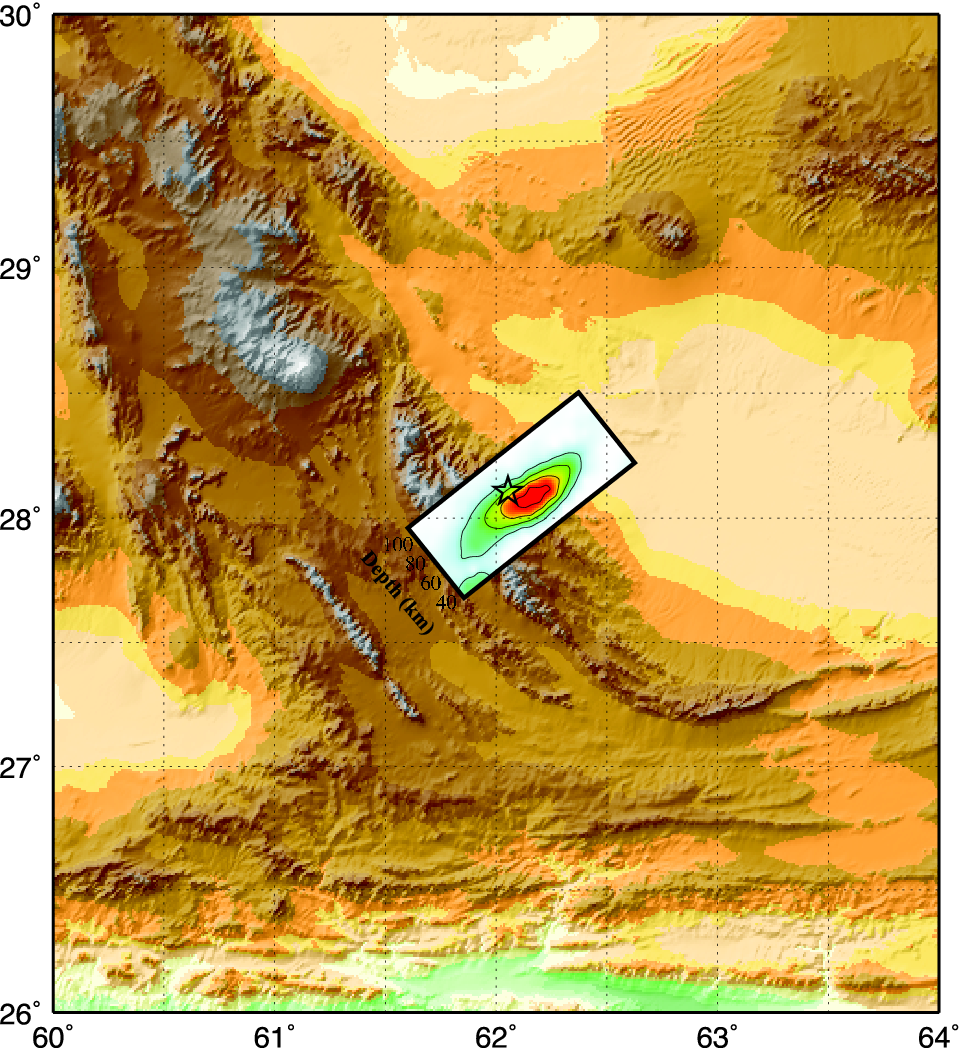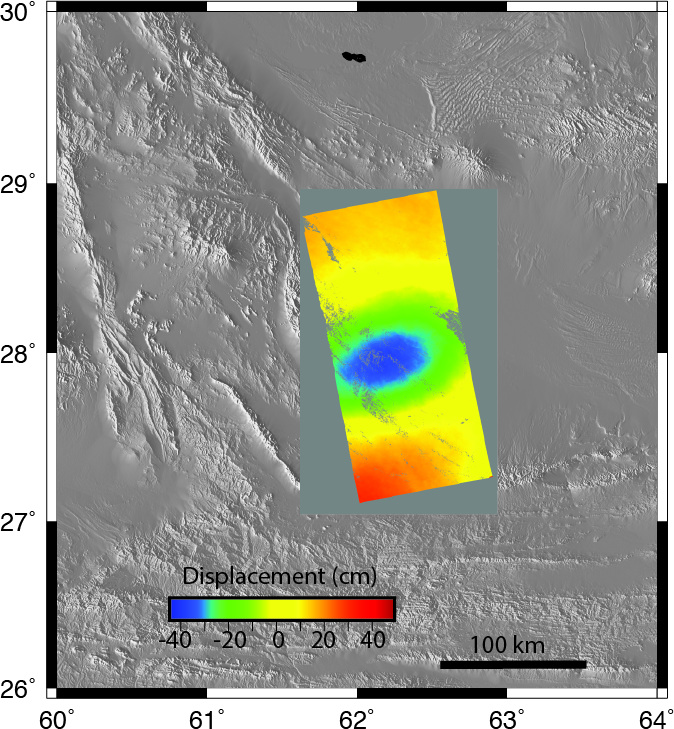### Test 1

The first test is whether the hstat96 give the same results as hsanal96 for a halfspace. Only the Z component predictions are compared. We will compare the results of using the GMT commands surface and xyz2grd. The shell script uses a finer sampling for surface.

 Vertical deformation (cm) for the halfspace model. The left  shows the result of using the analytic solution hsanal96 while the right column gives the solution using the propagator matrices and numerical wavenumber integration using hstat96.   The results are identical.  The upper and lower panels compare the xyz2grd and surface output respectively. Because the grid sampling interval was 0.25 degrees in latitude and longitude, for a 17 x 17 grid, the two plots are very similar.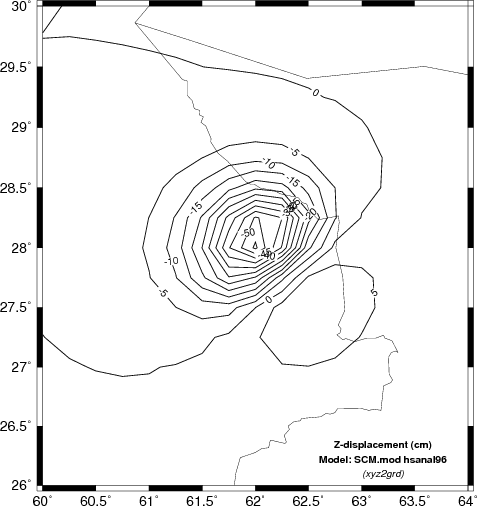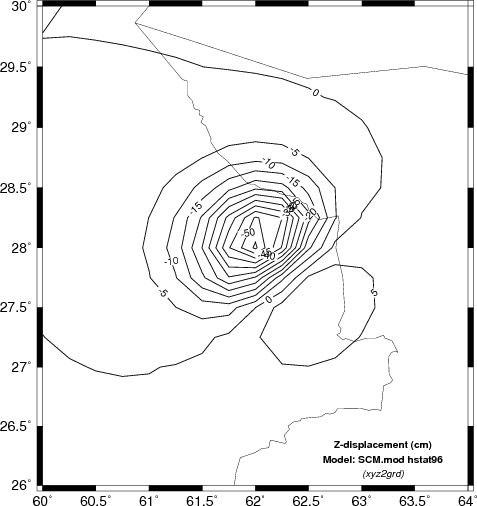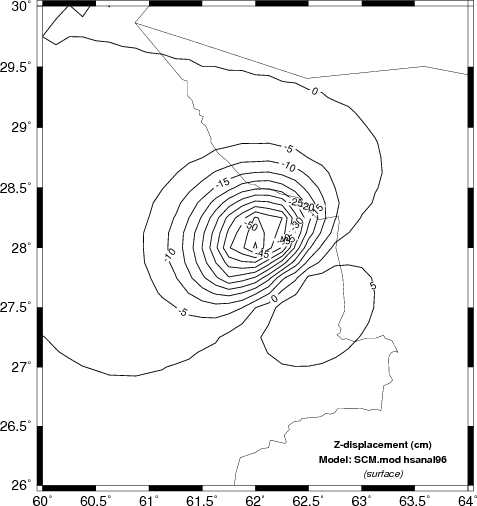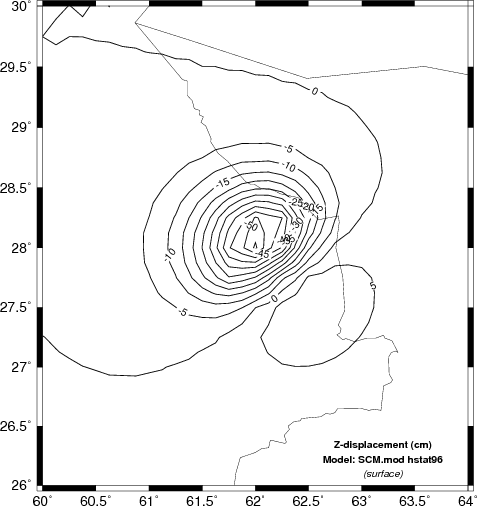### Coarser Sampling

To see the effect of sampling and the difference between the xyz2grd and surface output compare the following two figures to those above.  In this case the grid interval in latitude and longitude was 0.5 degrees, which yielded a 9x9 grid.  The program surface  smooths to a 0.25 degree grid. The difference in computational effort is roughly a factor of 4, e.g., (17x17)/(9x9), the number of grid locations.

 Vertical deformation (cm) determined using a coarser sampling grid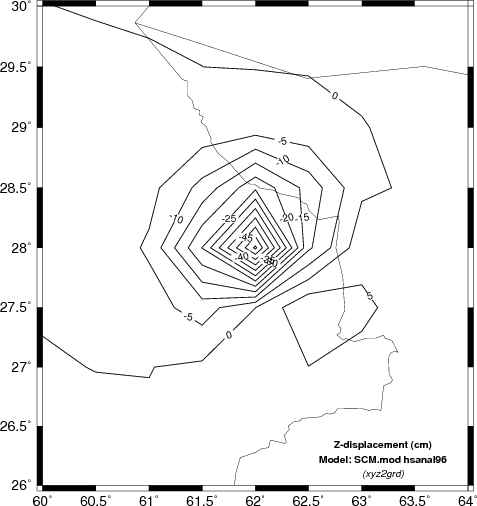### Test 2

The second test is to investigate the sensitivity of the finite fault solution to the velocity model used.
Here we will compare the computed displacements in the N (north) E (east) and Z (up directions). In the case of the Z comparison
we are able to compare the results to INSAR estimates.

 Comparison of vertical component deformation (cm) for the SCM, WUS and upper AK135 velocity models. These are compared to the INSAR image of the source region. Note that the maximum deformation for the synthetics seems to be about 10 km to the northwest of the depression indicated by the INSAR image. This offset is on the order of the teleseismic location error. The SCM model produces the largest surface displacements since the S-wave velocity at the source depth is much lower than for the other two models.``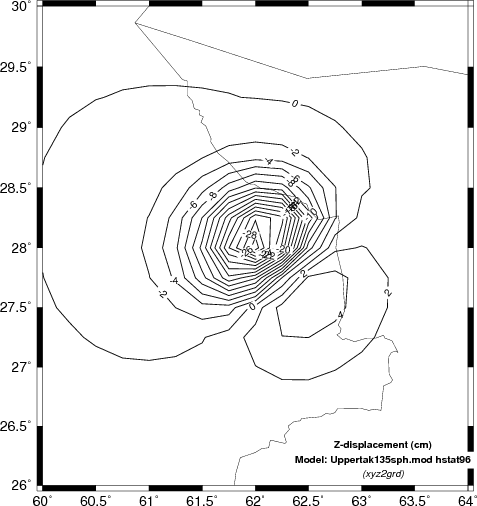`Comparison of north component deformation (cm) for the SCM, WUS and upper AK135 velocity models.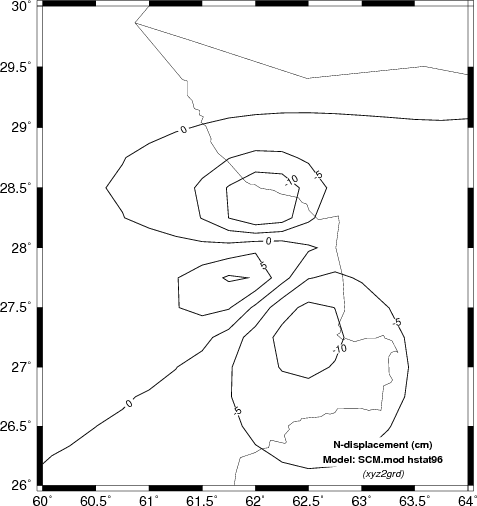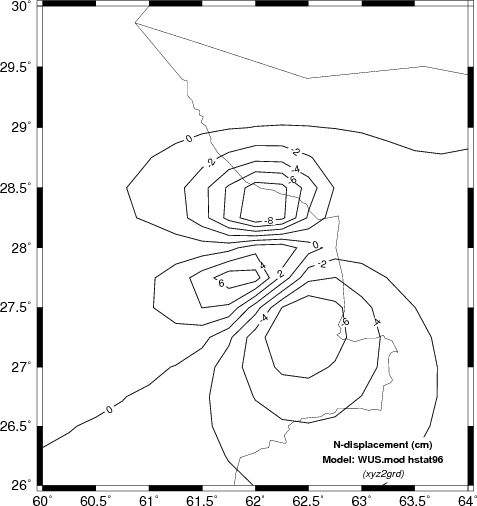Comparison of east component deformation (cm) for the SCM, WUS and upper AK135 velocity models.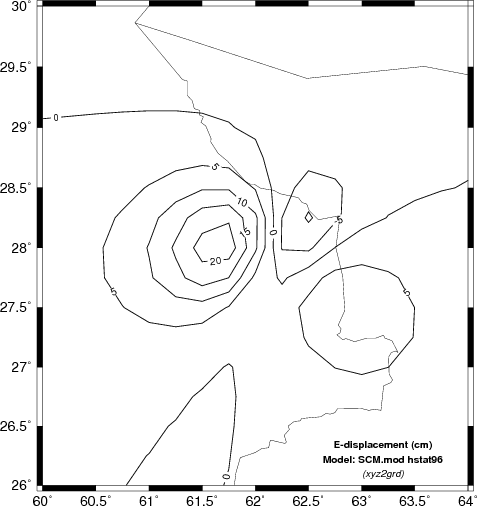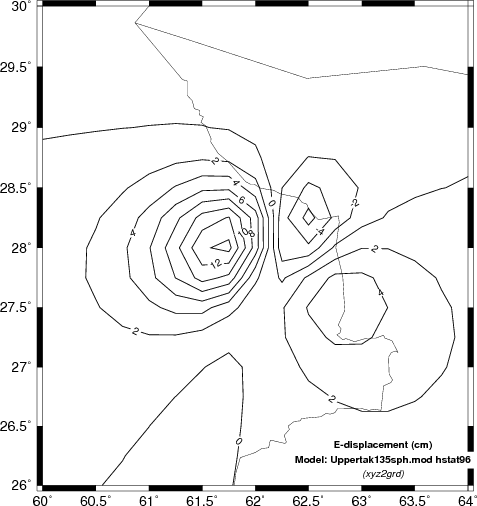Top of Page

## Discussion of solutions

The obvious difference among the predicted static displacements as a function of the velocity models is that the simple halfspace model predicts the highest amplitudes. This is not surprising since the theoretical amplitude is a function of the ratio (seismic moment)/rigidity. The velocity at the source depth in the simple model is the least, and hence the predicted amplitudes are the greatest.

Top of Page

## Pseudo time series

Since we are discussing the static deformation due to a rupturing finite fault, an interesting question concerns how the static deformation builds or when has the final static offset been achieved. As an approximation we assume here that the static offset is coincident with the first S-wave arrival. The link compares this assumption to a simulation that scales, shifts and adds complete regional seismograms. Comparison of displacement time histories.

Hopefully there may be some value in this presentation in addition to demonstrating how this can be done using these codes.

Top of Page

Last changed October 20, 2015

Avinash Nayak found an error in this documentation.